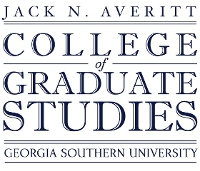## Electronic Theses and Dissertations

Spring 2020

#### Degree Name

Master of Science, Civil Engineering

#### Document Type and Release Option

Thesis (open access)

#### Department

Department of Civil Engineering and Construction

Saman Hedjazi

David Scott

Francisco Cubas

#### Abstract

This research investigates the relationship between early age ultrasonic pulse velocity and the compressive strength and dynamic modulus of steel, polypropylene, nylon, and glass fiber reinforced concrete. Previous studies prove that adding fibers to concrete alters the propagation of ultrasonic pulse velocity waves. Therefore, each type of fiber-reinforced concrete will have a unique relationship between ultrasonic pulse velocity and its compressive strength and dynamic modulus depending on fiber type, fiber volume fraction, water to cement ratio, and test age. To test this hypothesis, an experimental program comprising of one hundred eighty-nine 100 mm x 200 mm fiber reinforced concrete cylinders with varying fiber types, fiber volume fractions, and water-to-cement ratios will be tested using destructive and nondestructive test methods at the ages of 1, 3, 7, and 28 days. This research develops simple mathematical equations capable of predicting the compressive strength and dynamic modulus of different types of fiber-reinforced concrete based on early age ultrasonic pulse velocity. The equations for the prediction of the early age compressive strength of FRC had a coefficient of variation ranging from 6.4% to 14.6%. The equations for the prediction of the early age dynamic modulus of FRC had a coefficient of variation ranging from 3.3% to 9.2%. These equations can predict the compressive strength and dynamic modulus of multiple types of fiber reinforced concrete due to the incorporation of different fiber properties as variables in the equations.

1158465605

No

COinS